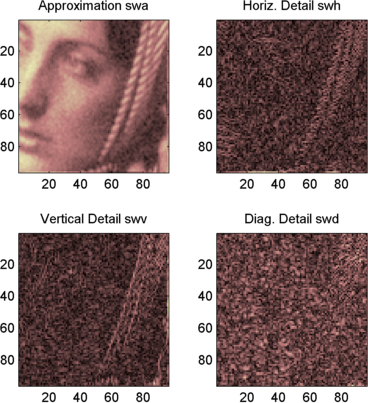## 2-D Stationary Wavelet Transform

This section takes you through the features of 2-D discrete stationary wavelet analysis using the Wavelet Toolbox™ software.

### Analysis-Decomposition Function

Function NamePurpose

`swt2`

Decomposition

### Synthesis-Reconstruction Function

Function NamePurpose

`iswt2`

Reconstruction

The stationary wavelet decomposition structure is more tractable than the wavelet one. So, the utilities useful for the wavelet case are not necessary for the Stationary Wavelet Transform (SWT).

In this section, you'll learn to

• Analyze an image

• Perform single-level and multilevel image decompositions and reconstructions

• Denoise an image

### 2-D Analysis

In this example, we'll show how you can use 2-D stationary wavelet analysis to denoise an image.

Note

Instead of using `image(I)` to visualize the image `I`, we use` image(wcodemat(I))`, which displays a rescaled version of `I` leading to a clearer presentation of the details and approximations (see the `wcodemat` reference page).

This example involves a image containing noise.

From the MATLAB® prompt, type

```load noiswom whos ```
NameSizeBytesClass
`X``96x96``73728``double array`
`map``255x3``6120``double array`

For the SWT, if a decomposition at level `k` is needed, `2^k` must divide evenly into `size(X,1)` and `size(X,2)`. If your original image is not of correct size, you can use the function `wextend` to extend it.

2. Perform a single-level Stationary Wavelet Decomposition.

Perform a single-level decomposition of the image using the `db1` wavelet. Type

```[swa,swh,swv,swd] = swt2(X,1,'db1'); ```

This generates the coefficients matrices of the level-one approximation (`swa`) and horizontal, vertical and diagonal details (`swh`, `swv`, and `swd`, respectively). Both are of size-the-image size. Type

```whos ```
NameSizeBytesClass
`X``96x96``73728``double array`
`map``255x3``6120``double array`
`swa``96x96``73728``double array`
`swh``96x96``73728``double array`
`swv``96x96``73728``double array`
`swd``96x96``73728``double array`
3. Display the coefficients of approximation and details.

To display the coefficients of approximation and details at level 1, type

```map = pink(size(map,1)); colormap(map) subplot(2,2,1), image(wcodemat(swa,192)); title('Approximation swa') subplot(2,2,2), image(wcodemat(swh,192)); title('Horiz. Detail swh') subplot(2,2,3), image(wcodemat(swv,192)); title('Vertical Detail swv') subplot(2,2,4), image(wcodemat(swd,192)); title('Diag. Detail swd'); ```4. Regenerate the image by Inverse Stationary Wavelet Transform.

To find the inverse transform, type

```A0 = iswt2(swa,swh,swv,swd,'db1'); ```

To check the perfect reconstruction, type

```err = max(max(abs(X-A0))) err = 1.1369e-13 ```
5. Construct and display approximation and details from the coefficients.

To construct the level 1 approximation and details (`A1`, `H1`, `V1` and `D1`) from the coefficients `swa`, `swh`, `swv` and `swd`, type

```nulcfs = zeros(size(swa)); A1 = iswt2(swa,nulcfs,nulcfs,nulcfs,'db1'); H1 = iswt2(nulcfs,swh,nulcfs,nulcfs,'db1'); V1 = iswt2(nulcfs,nulcfs,swv,nulcfs,'db1'); D1 = iswt2(nulcfs,nulcfs,nulcfs,swd,'db1'); ```

To display the approximation and details at level 1, type

```colormap(map) subplot(2,2,1), image(wcodemat(A1,192)); title('Approximation A1') subplot(2,2,2), image(wcodemat(H1,192)); title('Horiz. Detail H1') subplot(2,2,3), image(wcodemat(V1,192)); title('Vertical Detail V1') subplot(2,2,4), image(wcodemat(D1,192)); title('Diag. Detail D1') ```6. Perform a multilevel Stationary Wavelet Decomposition.

To perform a decomposition at level 3 of the image (again using the `db1` wavelet), type

```[swa,swh,swv,swd] = swt2(X,3,'db1'); ```

This generates the coefficients of the approximations at levels 1, 2, and 3 (`swa`) and the coefficients of the details (`swh`, `swv` and `swd`). Observe that the matrices `swa(:,:,i)`, `swh(:,:,i)`, `swv(:,:,i)`, and `swd(:,:,i)` for a given level `i` are of size-the-image size. Type

```clear A0 A1 D1 H1 V1 err nulcfs whos ```
NameSizeBytesClass
`X``96x96``73728``double array`
`map``255x3``6120``double array`
`swa``96x96x3``221184``double array`
`swh``96x96x3``221184``double array`
`swv``96x96x3``221184``double array`
`swd``96x96x3``221184``double array`
7. Display the coefficients of approximations and details.

To display the coefficients of approximations and details, type

```colormap(map) kp = 0; for i = 1:3 subplot(3,4,kp+1), image(wcodemat(swa(:,:,i),192)); title(['Approx. cfs level ',num2str(i)]) subplot(3,4,kp+2), image(wcodemat(swh(:,:,i),192)); title(['Horiz. Det. cfs level ',num2str(i)]) subplot(3,4,kp+3), image(wcodemat(swv(:,:,i),192)); title(['Vert. Det. cfs level ',num2str(i)]) subplot(3,4,kp+4), image(wcodemat(swd(:,:,i),192)); title(['Diag. Det. cfs level ',num2str(i)]) kp = kp + 4; end ```
8. Reconstruct approximation at Level 3 and details from coefficients.

To reconstruct the approximation at level 3, type

```mzero = zeros(size(swd)); A = mzero; A(:,:,3) = iswt2(swa,mzero,mzero,mzero,'db1'); ```

To reconstruct the details at levels 1, 2 and 3, type

```H = mzero; V = mzero; D = mzero; for i = 1:3 swcfs = mzero; swcfs(:,:,i) = swh(:,:,i); H(:,:,i) = iswt2(mzero,swcfs,mzero,mzero,'db1'); swcfs = mzero; swcfs(:,:,i) = swv(:,:,i); V(:,:,i) = iswt2(mzero,mzero,swcfs,mzero,'db1'); swcfs = mzero; swcfs(:,:,i) = swd(:,:,i); D(:,:,i) = iswt2(mzero,mzero,mzero,swcfs,'db1'); end ```
9. Reconstruct and display approximations at Levels 1, 2 from approximation at Level 3 and details at Levels 1, 2, and 3.

To reconstruct the approximations at levels 2 and 3, type

```A(:,:,2) = A(:,:,3) + H(:,:,3) + V(:,:,3) + D(:,:,3); A(:,:,1) = A(:,:,2) + H(:,:,2) + V(:,:,2) + D(:,:,2); ```

To display the approximations and details at levels 1, 2, and 3, type

```colormap(map) kp = 0; for i = 1:3 subplot(3,4,kp+1), image(wcodemat(A(:,:,i),192)); title(['Approx. level ',num2str(i)]) subplot(3,4,kp+2), image(wcodemat(H(:,:,i),192)); title(['Horiz. Det. level ',num2str(i)]) subplot(3,4,kp+3), image(wcodemat(V(:,:,i),192)); title(['Vert. Det. level ',num2str(i)]) subplot(3,4,kp+4), image(wcodemat(D(:,:,i),192)); title(['Diag. Det. level ',num2str(i)]) kp = kp + 4; end ```
10. Remove noise by thresholding.

To denoise an image, use the `ddencmp` function to find the threshold value, use the `wthresh` function to perform the actual thresholding of the detail coefficients, and then use the `iswt2` function to obtain the denoised image.

```thr = 44.5; sorh = 's'; dswh = wthresh(swh,sorh,thr); dswv = wthresh(swv,sorh,thr); dswd = wthresh(swd,sorh,thr); clean = iswt2(swa,dswh,dswv,dswd,'db1'); ```

To display both the original and denoised images, type

```colormap(map) subplot(1,2,1), image(wcodemat(X,192)); title('Original image') subplot(1,2,2), image(wcodemat(clean,192)); title('denoised image') ```A second syntax can be used for the `swt2` and `iswt2` functions, giving the same results:

```lev= 4; swc = swt2(X,lev,'db1'); swcden = swc; swcden(:,:,1:end-1) = wthresh(swcden(:,:,1:end-1),sorh,thr); clean = iswt2(swcden,'db1'); ```

You obtain the same plot by using the plot commands in step 9 above.